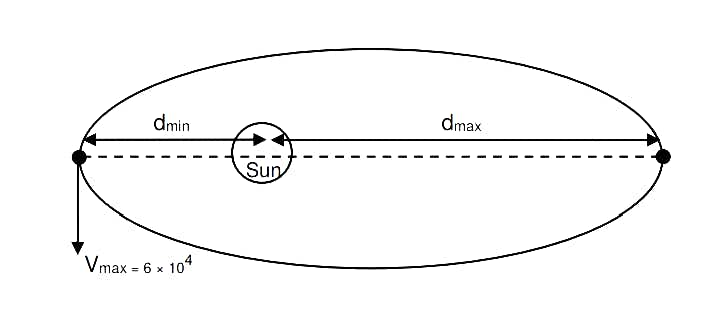+1 vote
17 views

The maximum and minimum distances of comet from the Sun are 1.6 × 1012 m and 8.0 × 1010 m respectively If the speed of the comet at the nearest point is 6 × 104 ms–1 , the speed at the farthest point is :

(1) 3.0 × 103 m/s (2) 4.5 × 103 m/s (3) 1.5 × 103 m/s (4) 6.0 × 103 m/s

in Physics
reopened | 17 views

## 1 Answer

0 votes
Best answer

The correct answer is option A) 3.0×103 m/s

Explanation::$$L=mv_1r_1=mv_2r_2$$

$$V_2={ v_1r_1\over r_2}$$

$$=v_1{ 8×10^{10}\over 160×10^{10}}$$

$$={6×10^4\over 20}$$

$$=3000 m/s$$

by (4.0k points)